# GED Math : Statistics

## Example Questions

### Example Question #3 : Median

Philip went to a family reunion this weekend and met his extended cousins, whose ages were the following:

10, 21, 7, 8, 19, 20, 8, 15, 17, and 13.

What is the median age?

10

14

17

13

8

14

Explanation:

The median is the middle number in a data set.

To find the median, rearrange the numbers in ascending order and find the middle number:

Given: 10, 21, 7, 8, 19, 20, 8, 15, 17, 13.

In order: 7, 8, 8, 10, 13, 15, 17, 19, 20, 21.

Since the data set has 10 numbers, which is even, the median is the average of the two middle numbers. The two numbers in the middle of this data set are 13 and 15. Their average, or the number in between them, is 14.

Therefore the median age is 14.

### Example Question #6 : Median

Use the following data set of test scores to answer the question: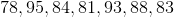Find the median.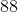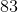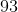Explanation:

To find the median score, we will first arrange the numbers in ascending order. Then, we will find the number in the middle of the set.

So, given the setwe will arrange the numbers in ascending order (from smallest to largest). So, we get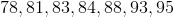Now, we will find the number in the middle.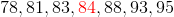We can see that it is 84.

Therefore, the median score of the data set is 84.

### Example Question #7 : Median

A Science class took an exam. Here are the scores of 9 students: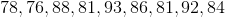Find the median score.Explanation:

To find the median score, we will first arrange the scores in ascending order. Then, we will find the score in the middle of the set.

So, given the setwe will first arrange them in ascending order (from smallest to largest). So, we get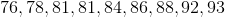Now, we will find the score in the middle of the set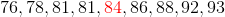we can see that it is 84.

Therefore, the median score is 84.

### Example Question #8 : Median

A class took a Math exam.  Here are the test scores of 11 students.Find the median.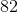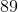Explanation:

To find the median of a data set, we will first arrange the numbers in ascending order. Then, we will find the number in the middle of the set. So, given the set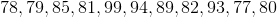we will arrange the numbers in ascending order (from smallest to largest). We get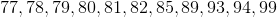Now, we will find the number in the middle of the set.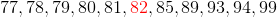Therefore, the median of the data set is 82.

### Example Question #9 : Median

Solve for the median: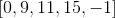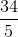Explanation:

Reorder the numbers from least to greatest.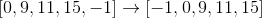In an odd set of data, the median is the central number of this data set.

The answer is:### Example Question #10 : Median

Identify the median: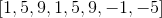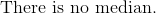Explanation:

Reorder all the numbers in chronological order.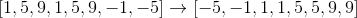The median of an even set of numbers is the average of the central two numbers.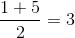The median is:### Example Question #11 : Median

A class took a Math exam. Here are the test scores of 9 students.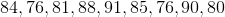Find the median.Explanation:

To find the median of a data set, we will first arrange the numbers in ascending order. Then, we will find the number in the middle of the set. So, given the setwe will arrange the numbers in ascending order (from smallest to largest). We get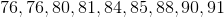Now, we will find the number in the middle of the set.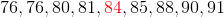Therefore, the median of the data set is 84.

### Example Question #12 : Median

Determine the median of the numbers: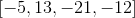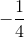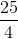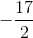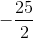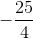Explanation:

Reorder the numbers from least to greatest.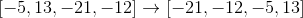The median is the average of the central numbers of an ordered set of numbers.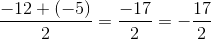The answer is:### Example Question #13 : Median

Determine the median: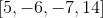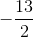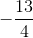Explanation:

Rewrite the data set from least to greatest.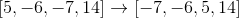Average the central two numbers.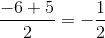The answer is:### Example Question #101 : Statistics

Find the median: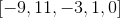Explanation:

Reorder the numbers in the data set from least to greatest.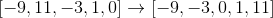The median is the central number for an odd set of numbers.

The answer is:### All GED Math Resources